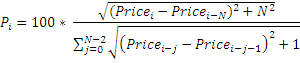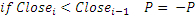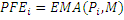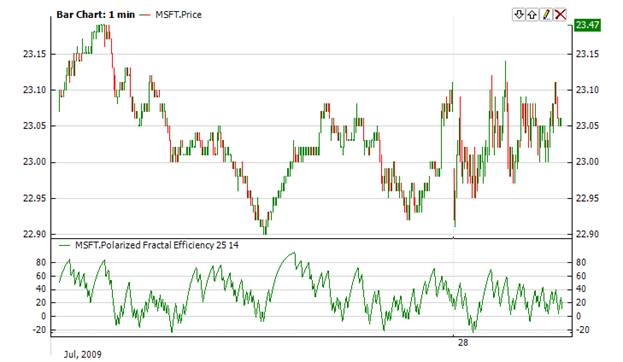﻿Polarized Fractal Efficiency

# Polarized Fractal Efficiency

The Polarized Fractal Efficiency (Pfe) indicator uses fractal geometry to determine how efficiently the price is moving. The indicator is used for calculation of how trendy or overloaded the price action is. Pfe below zero - means that the trend is down. Pfe above 0 - indicates that the trend is up. The higher the value of indicator, the more pronounced the upward movement. Value near zero points to a balance between demand and supply.Market Signals

The buy signal is a reversal in the direction of the indicator and its movement from the minimum to zero. Signal to close the position occurs if the value of the indicator reaches its peak above zero.  Indicator movement from peak to zero gives a sell signal. Any short position should be covered after the formation of a new minimum of the value of indicator.CalculationWhere N - period of indicator, M - smoothing period.Chart ExampleImplementation and Usage

To initialize Pfe use one of the following constructors:

Pfe – sets default values: period = 14, smoothingPeriod = 3

Pfe(Int32, Int32) – sets values for indicator

Use

PFE - property to get current valueExample
C#
``` 1// Create new instance
2Pfe pfe = new Pfe(28, 9);
3
4// Number of stored values
5pfe.HistoryCapacity = 2;
6